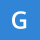Home Communities
IT Knowledge
Inspiration
Languages
EN

# Python - get current datetime

0 points
Created by:Gaia-Kirby
390

In this article, we would like to show you how get current datetime in Python.

To work with date objects, you need to import the datetime module using:

``import datetime``

## 1. Get current datetime

In this example, we use `datetime.datetime.now()` to get the current datetime.

``````import datetime

result = datetime.datetime.now()

print(result)  # 2021-08-19 20:27:43.361352 (current datetime)``````

Output:

``2021-08-19 20:27:43.361352``

## 2. Get year / month / day

In this example, we get year, month and day from current datetime.

``````from datetime import datetime

now = datetime.now()  # current datetime

print(now.year)
print(now.month)
print(now.day)``````

Example output:

``````2021
8
19``````

## 3. Get hour / minute / second

In this example, we get hour, minute and second from current datetime.

``````from datetime import datetime

now = datetime.now()  # current datetime

print(now.hour)
print(now.minute)
print(now.second)``````

Example output:

``````21
3
40``````